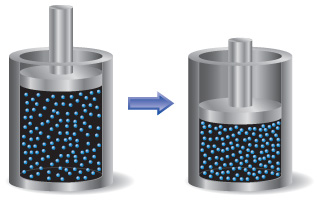# Problem: A sample of gas is contained in a cylinder-and-piston arrangement. It undergoes the change in state shown in the drawing. Assume first that the cylinder and piston are perfect thermal insulators that do not allow heat to be transferred.What is the sign of w for the state change?

###### FREE Expert Solution

We’re being asked to identify the sign of work (w). given the illustration.

Recall that work (w) is given by:

$\overline{){\mathbf{w}}{\mathbf{=}}{\mathbf{-}}{\mathbf{P\Delta V}}}$

where:

P = pressure (in atm)

ΔV = Vfinal – Vinitial = change in volume (in L)

81% (329 ratings)###### Problem Details

A sample of gas is contained in a cylinder-and-piston arrangement. It undergoes the change in state shown in the drawing. Assume first that the cylinder and piston are perfect thermal insulators that do not allow heat to be transferred.What is the sign of w for the state change?